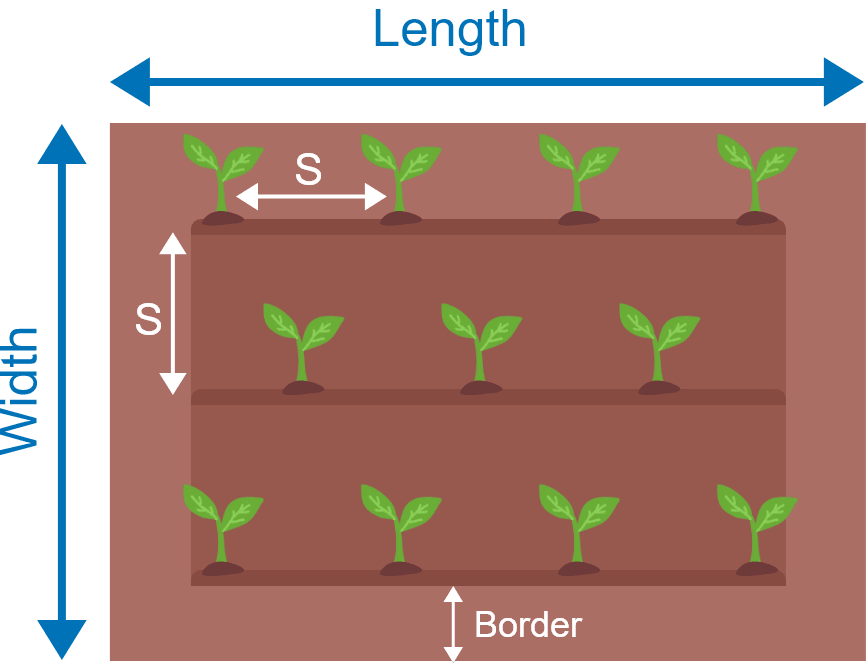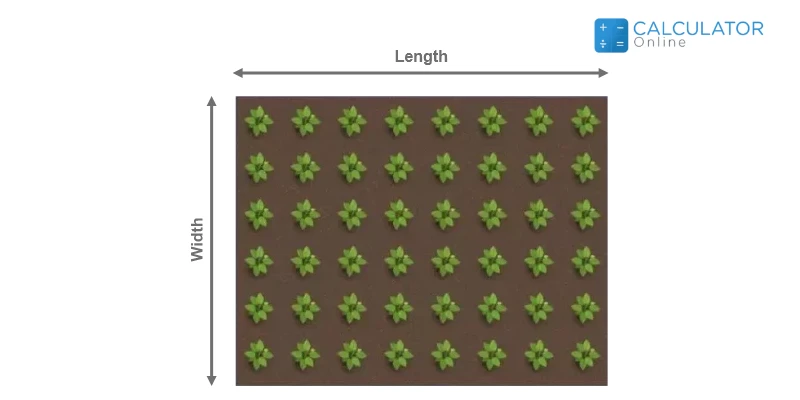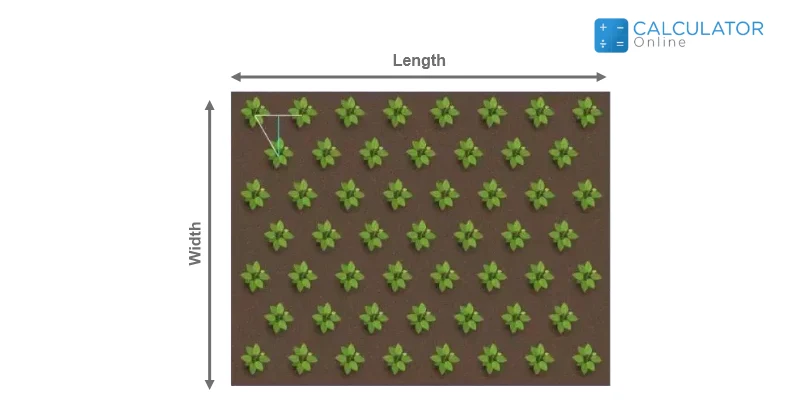Uh Oh! It seems you’re using an Ad blocker!

We always struggled to serve you with the best online calculations, thus, there's a humble request to either disable the AD blocker or go with premium plans to use the AD-Free version for calculators.

Or# Plant Spacing Calculator

Planting bed:

Plant Grid:

Number of Rows:Area length:

Area width:

I want to know

Border:

Length of hedge:

Plant Spacing:

Row Spacing :

Total Plant:

Total Rows:

Plant Cost:

No. of Plants:

Cost per Plant:

\$

Table of Content

Get the Widget!

Add this calculator to your site and lets users to perform easy calculations.

Feedback

How easy was it to use our calculator? Did you face any problem, tell us!

Our Free Plant Spacing Calculator will instantly calculate plant spacing that will help you navigate the total number of plants within a certain land piece. This would help us to estimate the number of plants or trees per square unit area.

## Why Calculate Plant Spacing?

The plant spacing is necessary for proper maintenance and estimating per-acre Cultivation Costs. The other thing is the harvesting equipment and the overall fertilizer cost. You need to understand there is an appropriate space required for each plant to grow properly. The Plant Spacing Calculator assists us to determine the proper spacing between the plants in our field for evergreen cultivation.

### How to Measure Plant Spacing?

You can measure the total number of plants in a unit area and the appropriate spacing by using the following formula

Area of field= Length × Width (Units in Metric or Imperial Units)

The number of plants in the field or garden can be measured by the square pattern and triangular pattern of the field. The plant calculator estimates the number of plants both by the square spacing methodology and also by the triangular pattern.

### Square Spacing Formula:

The garden spacing can be measured by the square spacing method. It is a simple method of measuring the appropriate spacing between the plantations in a given perimeter of the field.Plants per Row = Length/ Plant Spacing

Plants per Column = Length/ Plant Spacing

Total number of Plants = Plants per Row × Plants per Column

You can also measure the square spacing of plants by another formula:

Total Number of Plants = [Area of Garden/(Plant Spacing)^2]

### Triangular Spacing:

The other method is Triangular spacing, and you need to place the plants in the corners of an equilateral triangle. Normally the plants are at 60 degrees to each other in the equilateral triangle. You can use the arborvitae spacing calculator to measure the triangular spacing between the plants.Row Spacing = Plant Spacing × 0.866

For odd rows (1,3,5,7, etc):

Plants Number (O) = [Length/Plant Spacing]

For even rows (2,4,6,8, etc):

Plants Number (E) = [Length-Plant Spacing×0.5/Plant Spacing]

Total Rows = [(Width – Plants Spacing)/Row Spacing]+1

For finding the even number of Rows (2,4,6,8, etc)

Row (E) = [Total number of Rows/2]

The number of odd Rows (1,3,5,7, etc)

Row (O) = Total number of Rows-Row (E)

The total number of plants is given below:

Total Number of Plants =  Plants Number (E)× Row (E) +  Plants Number (O)× Row (O)

You can adjust the number of plants in your garden by the garden spacing calculator.

### Plant Spacing Chart:

The plant spacing chart for various values is given below:

Area Length Area Width Area Size Plant Spacing Plant Density Plant Grid Total Plant
100 ft 50 ft 5000 ft2 6 ft 0.02778 17×8 136
50 m 20 m 1000 m2 0.5 m 4 101×41 4141
100 yds 50 yds 5000 yds 2 3 m 0.0929 16×31 496

These are the values checked by the planting calculator and computed against various values.

### How to Use Plant Spacing Calculator?

For precise measurements of the number of plants in your garden by the plant spacing calculator, just follow the steps given below:

Input:

• Choose the Planting Bed
• Select the Plant Grid
• Enter Field’s Area, Length, and Width
• Enter the Border width and Plant Spacing
• Enter the Number of Plants and Cost/Plant
• Hit the calculate Button

Output:

• The Total Number of Plants
• The Total Plantation Cost

### References:

From the source of homeguides.sfgate.com: Vegetable Planting,Plant & Row Spacing

From the source of knowledgebank.irri.org: Spacing of Plants, Variety